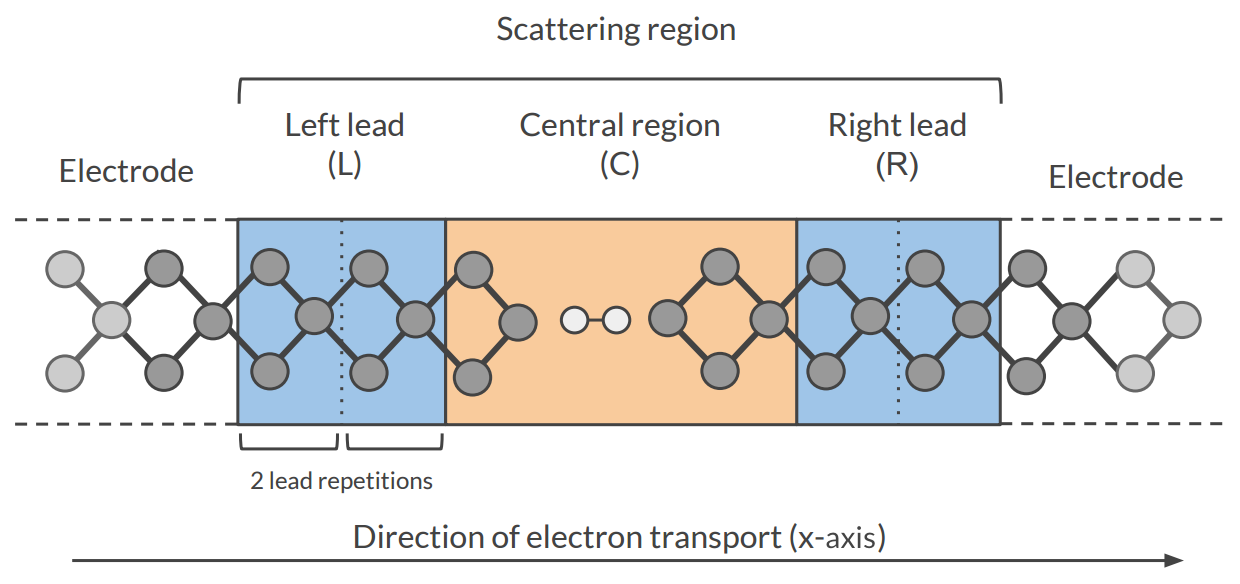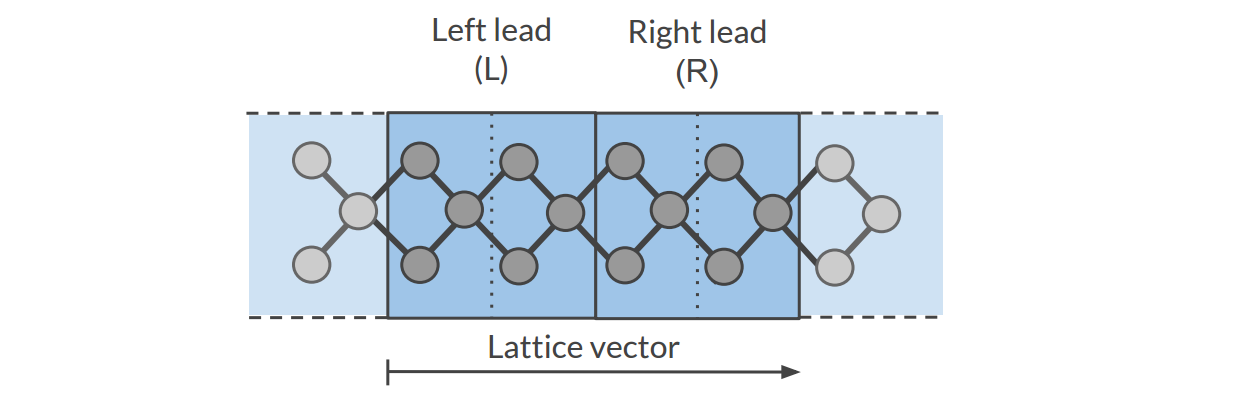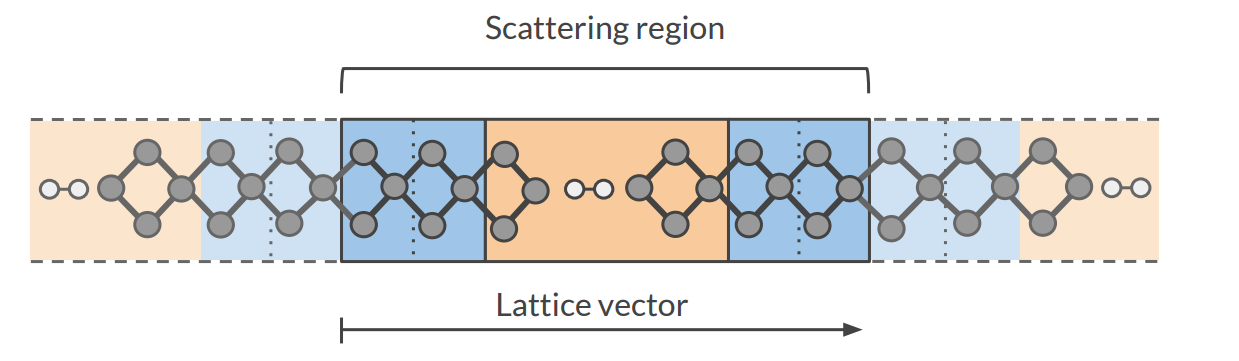# Electronic transport (NEGF)¶

## Transport with NEGF in a nutshell¶

The Non-Equilibrium Green’s Functions formalism (NEGF) is a theoretical framework for modeling electron transport through nano-scale devices. Electron transport is treated as a one-dimensional coherent scattering process in the “scattering region” for electrons coming in from the electrodes:Our goal is to compute the transmission function $$T(E)$$, which describes the rate at which electrons of energy $$E$$ are transferred from the left electrode to the right electrode by propagating through the scattering region. From the transmission function we can calculate the electric current for given Bias Voltage $$V$$ applied between the electrodes:

$I(V) = \frac{2e}{h} \int_{-\infty}^\infty T(E) \left( f(E - \mu_L) - f(E - \mu_R) \right) dE$

where $$f(E)$$ is the Fermi-Dirac distribution function for a given temperature, and $$\mu_L$$ ($$\mu_R$$) is $$\epsilon_F + eV/2$$ ($$\epsilon_F - eV/2$$), $$\epsilon_F$$ being the Fermi energy of the electrodes.

The transmission function $$T(E)$$ can be computed from the Green’s function of our system.

The Green’s function $$G(E)$$ of the scattering region is obtained solving the following equation:

$(E S - H) G(E) = I$

where $$S$$ is the overlap matrix, $$H$$ is the Hamiltonian and $$I$$ is the identity matrix. The Hamiltonian is composed as follows (L, C and R denote the left lead, the central region and the right lead respectively):

$\begin{split}H = \left( \begin{array}{ccc} H_L + \Sigma_L & H_{LC} & 0 \\ H_{LC} & H_C & H_{RC} \\ 0 & H_{RC} & H_R + \Sigma_R \end{array} \right)\end{split}$

The two self-energies $$\Sigma_L$$ and $$\Sigma_R$$ model the two semi-infinite electrodes.

The transmission function $$T(E)$$ can be calculated from the Green’s function $$G(E)$$ and the so-called coupling matrices $$\Gamma_L(E)$$ and $$\Gamma_R(E)$$ (which are related to $$\Sigma_L$$ and $$\Sigma_R$$):

$T(E) = Tr[G(E) \Gamma_R(E) G(E) \Gamma_L(E)]$

PhD Thesis of Mahdi Ghorbani-Asl (DFTB-NEGF developer)

## Simulations work flow¶

The computation of the transmission function $$T(E)$$ within the DFTB-NEGF formalisms requires three individual simulations.

Tip

Use ADFInput (GUI) to set up your DFTB-NEGF calculation (see the DFTB-NEGF GUI tutorial)

A 1D-periodic DFTB calculation of the leads (StoreMatrices: yes, KSpace sampling 13):The Hamiltonian matrices $$H_L$$ and $$H_{R}$$ and the Fermi energy of the electrode $$\epsilon_F$$ are computed in this calculation ($$H_L$$, $$H_{R}$$ and $$H_{LR}$$ are also used to compute the surface Green’s functions $$g_L$$ and $$g_R$$ of the semi-infinite electrodes).

2): DFTB scattering-region calculation

A a 1D-periodic DFTB calculation of the scattering region (StoreMatrices: yes, gamma-only, i.e., no KSpace sampling):The Hamiltonian matrices $$H_{LC}$$ and $$H_{RC}$$ and $$H_{C}$$ are computed in this calculation.

3): Conductance calculation
The Conductance program computes the NEGF transmission function $$T(E)$$ using the Hamiltonians and Overlap matrices from the previous two DFTB calculations.

## Conductance input options¶

The Conductance program computes the transmission function using the NEGF approach. This is the input structure of the conductance program:

\$ADFBIN/conductance <<EOF > conductance.out

EnergyGrid
Min value
Max value
Num value

Files
Scattering  /path/DFTB_scattering_filename.rkf
End

Technical
Eta                   value
SetOffDiagonalToZero  [True|False]
End

end input
EOF

EnergyGrid
Type: Block Energy grid for Transmission Function
Max
Type: Float 5.0 eV Max Energy (relative to Fermi energy)
Min
Type: Float -5.0 eV Min energy (relative to Fermi energy)
Num
Type: Integer 200 Number of energy values in which the interval Min-Max is subdivided
Technical
Type: Block options describing technical parts of the calculation
Eta
Type: Float 1e-05 To avoid poles of the Green’s function, a small imaginary number is added to the energy
overwriteLeads
Type: Bool True If true, Hamiltonians H_L and H_R are taken from the DFTB-leads calculation. If False, they are taken from the DFTB scattering-region calculation
setOffDiagonalToZero
Type: Bool True If true, H_LR and S_LR are explicitly set to zero. If False, they are taken from the DFTB scattering-region calculation.
Files
Type: Block path of files
Leads
Type: String Path (either absolute or relative) of the lead results file
Scattering
Type: String Path (either absolute or relative) of the scattering region results

## Miscellaneous remarks on DFTB-NEGF¶

• You should make sure that your results are converged with respect to the number of lead repetitions; the results should not change significantly if you increase the number of lead repetitions.
• It’s good practice to include at least one lead repetition in the central region.
• The transmission function is computed at zero bias voltage. The zero-bias transmission function is then used for computing the electric current for non-zero bias voltage.